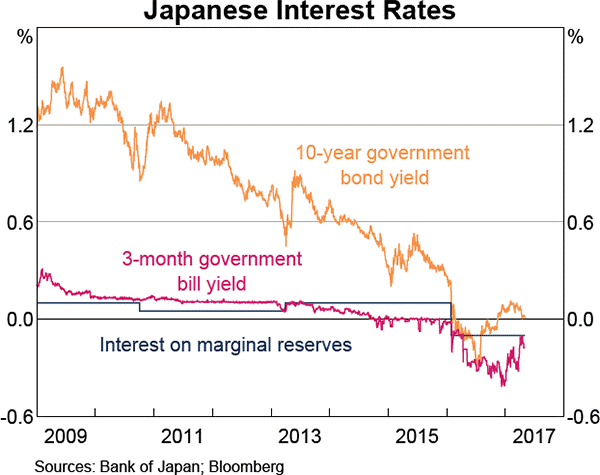# Fight Finance

#### CoursesTagsRandomAllRecentScores

A project has the following cash flows:

 Project Cash Flows Time (yrs) Cash flow ($) 0 -400 1 200 2 250 What is the Profitability Index (PI) of the project? Assume that the cash flows shown in the table are paid all at once at the given point in time. The required return is 10% pa, given as an effective annual rate. A project has the following cash flows:  Project Cash Flows Time (yrs) Cash flow ($) 0 -90 1 30 2 105

The required return of a project is 10%, given as an effective annual rate. Assume that the cash flows shown in the table are paid all at once at the given point in time.

What is the Profitability Index (PI) of the project?

Find World Bar's Cash Flow From Assets (CFFA), also known as Free Cash Flow to the Firm (FCFF), over the year ending 30th June 2013.

 World Bar Income Statement for year ending 30th June 2013 $m Sales 300 COGS 150 Operating expense 50 Depreciation 40 Interest expense 10 Taxable income 50 Tax at 30% 15 Net income 35  World Bar Balance Sheet as at 30th June 2013 2012$m $m Assets Current assets 200 230 PPE Cost 400 400 Accumul. depr. 75 35 Carrying amount 325 365 Total assets 525 595 Liabilities Current liabilities 150 205 Non-current liabilities 235 250 Owners' equity Retained earnings 100 100 Contributed equity 40 40 Total L and OE 525 595 Note: all figures above and below are given in millions of dollars ($m).

You have $100,000 in the bank. The bank pays interest at 10% pa, given as an effective annual rate. You wish to consume an equal amount now (t=0), in one year (t=1) and in two years (t=2), and still have$50,000 in the bank after that (t=2).

How much can you consume at each time?

A timing option is best modeled as a or option?

The perpetuity with growth formula is:

$$P_0= \dfrac{C_1}{r-g}$$

Which of the following is NOT equal to the total required return (r)?

Will the price of an out-of-the-money put option on equity or if the standard deviation of returns (risk) of the underlying shares becomes higher?

In Australia in the 1980's, inflation was around 8% pa, and residential mortgage loan interest rates were around 14%.

In 2013, inflation was around 2.5% pa, and residential mortgage loan interest rates were around 4.5%.

If a person can afford constant mortgage loan payments of \$2,000 per month, how much more can they borrow when interest rates are 4.5% pa compared with 14.0% pa?

Give your answer as a proportional increase over the amount you could borrow when interest rates were high $(V_\text{high rates})$, so:

$$\text{Proportional increase} = \dfrac{V_\text{low rates}-V_\text{high rates}}{V_\text{high rates}}$$

Assume that:

• Interest rates are expected to be constant over the life of the loan.
• Loans are interest-only and have a life of 30 years.
• Mortgage loan payments are made every month in arrears and all interest rates are given as annualised percentage rates (APR's) compounding per month.

Refer to the below graph when answering the questions.Which of the following statements is NOT correct?

Question 923  omitted variable bias, CAPM, single factor model, single index model, no explanation

Capital Asset Pricing Model (CAPM) and the Single Index Model (SIM) are single factor models whose only risk factor is the market portfolio’s return. Say a Taxi company and an Umbrella company are influenced by two factors, the market portfolio return and rainfall. When it rains, both the Taxi and Umbrella companies’ stock prices do well. When there’s no rain, both do poorly. Assume that rainfall risk is a systematic risk that cannot be diversified and that rainfall has zero correlation with the market portfolio’s returns.

Which of the following statements about these two stocks is NOT correct?

The CAPM and SIM: